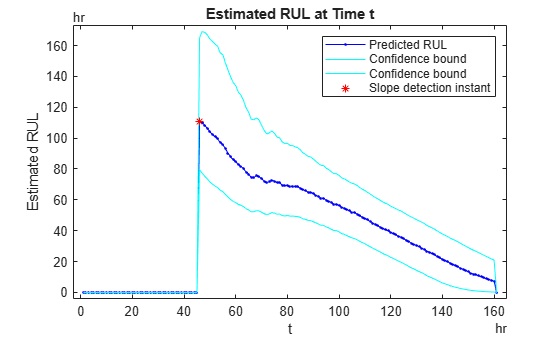# Update RUL Prediction as Data Arrives

This example shows how to update an RUL prediction as new data arrives from a machine under test. In the example, you use an ensemble of training data to train an RUL model. You then loop through a sequence of test data from a single machine, updating the RUL prediction for each new data point. The example shows the evolution of the RUL prediction as new data becomes available.

This example uses exponentialDegradationModel. For degradation RUL models, when a new data point becomes available, you must first update the degradation model before predicting a new RUL value. For other RUL model types, skip this update step.

### Data for Training and Prediction

Load the data for this example, which consists of two variables, TestData and TrainingData.

load UpdateRULExampleData

TestData is a table containing the value of some condition indicator, Condition, recorded every hour, as the first few entries show.

head(TestData,5)
ans=5×2 table Time Condition ____ _________ 1 1.0552 2 1.2013 3 0.79781 4 1.09 5 1.0324 

TrainingData is a cell array of tables having the same variables as TestData. Each cell in TrainingData represents the evolution to failure of the condition indicator Condition over the lifetime of one machine in the ensemble.

### Train Prediction Model

Use TrainingData to train an exponentialDegradationModel model for RUL prediction. The fit command estimates a prior for the model's parameters based on the historical records in TrainingData. The Prior property of the trained model contains the model parameters Theta, Beta, and Rho. (For details of these model parameters, see exponentialDegradationModel.)

mdl = exponentialDegradationModel('LifeTimeUnit',"hours"); fit(mdl,TrainingData,"Time","Condition") mdl.Prior
ans = struct with fields: Theta: 0.6389 ThetaVariance: 0.0661 Beta: 0.0583 BetaVariance: 1.8383e-04 Rho: -0.3129 

Degradation models are most reliable for degradation tracking after an initial slope emerges in the condition-indicator measurements. Set the slope detection level at 0.1 to tell the model not to make RUL predictions until that slope is reached. (When you know in advance that the measurements are for a component whose degradation has already started, you can disable slope detection by setting mdl.SlopeDetectionLevel = [].)

mdl.SlopeDetectionLevel = 0.1;

### Predict RUL at Each Time Step

Define a threshold condition indicator value that indicates the end of life of a machine. The RUL is the predicted time left before the condition indicator for the test machine reaches this threshold value.

threshold = 400;

For RUL prediction, assume that TestData begins at time t = 1 hour, and a new data sample becomes available every hour. In general, you can predict a new RUL value with each new data point. For the degradation model of this example, loop through TestData and update the model with each new data point using the update command. Then, check whether the model detects a sufficient change in slope for reliable RUL prediction. If it does, predict a new RUL value using the predictRUL command. To observe the evolution of the estimation, store the estimated RUL values and the associated confidence intervals in the vectors EstRUL and CI, respectively. Similarly, store the model parameters in the array ModelParameters.

N = height(TestData); EstRUL = hours(zeros(N,1)); CI = hours(zeros(N,2)); ModelParameters = zeros(N,3); for t = 1:N CurrentDataSample = TestData(t,:); update(mdl,CurrentDataSample) ModelParameters(t,:) = [mdl.Theta mdl.Beta mdl.Rho]; % Compute RUL only if the data indicates a change in slope. if ~isempty(mdl.SlopeDetectionInstant) [EstRUL(t),CI(t,:)] = predictRUL(mdl,CurrentDataSample,threshold); end end

Plot the trajectories of the estimated model-parameter values. The values change rapidly after a slope is detected in the degradation data. They tend to converge as more data points become available.

Time = hours(1:N)'; tStart = mdl.SlopeDetectionInstant; % slope detection time instant plot(Time,ModelParameters); hold on plot([tStart, tStart],[-1,2],'k--') legend({'\theta(t)','\beta(t)','\rho(t)','Slope detection instant'},'Location','best') hold offPlot the predicted RUL to observe its evolution as more degradation data becomes available. There is no new estimated RUL value until a slope is detected in the degradation data. After that, the predicted RUL decreases over time, as expected. predictRUL computes a statistical distribution of RUL values. The confidence bounds on the predicted RUL become narrower over time.

plot(Time,EstRUL,'b.-',Time,CI,'c',tStart,EstRUL(hours(tStart)),'r*') title('Estimated RUL at Time t') xlabel('t') ylabel('Estimated RUL') legend({'Predicted RUL','Confidence bound','Confidence bound','Slope detection instant'})